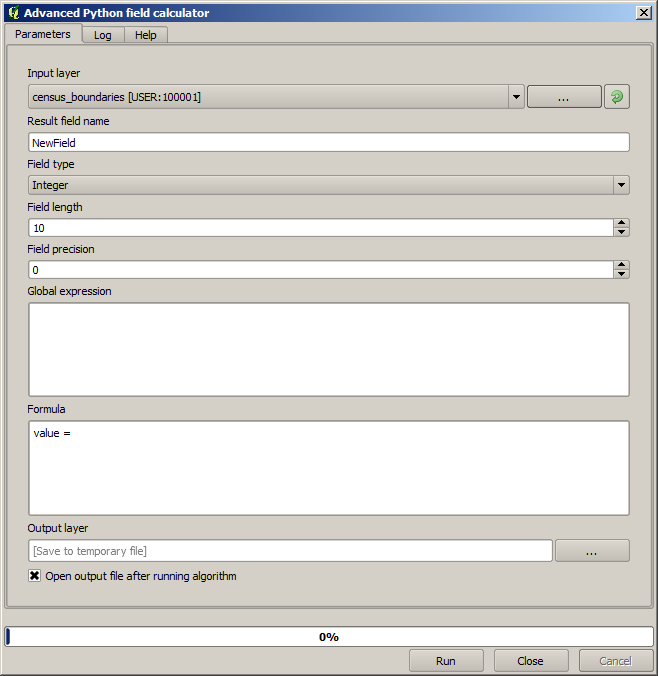### Outdated version of the documentation. Find the latest one here.

Nota

In this lesson we will see how to add new attributes to a vector layer based on a mathematical expression, using the vector calculator

We already know how to use the raster calculator to create new raster layers usig mathematical expressions. A similar algorithm is available for vector layer, and generates a new layer with the same attributes of the input layer, plus an additional on with the result of the expression entered. The algorithm is called Field calculator and has the following parameters dialog.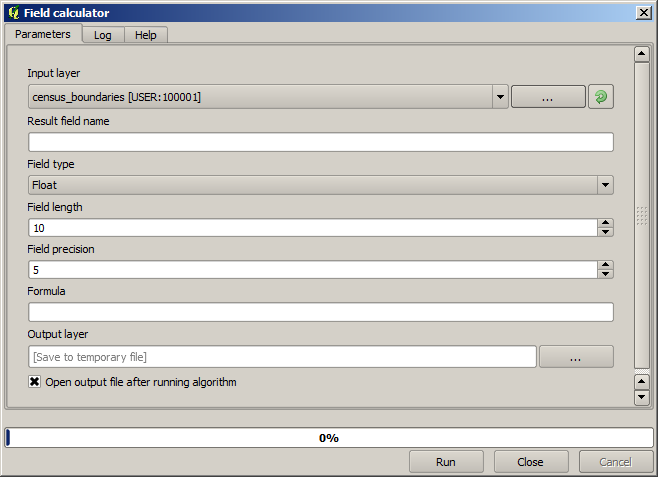Nota

Em novas versões a interface de processamento foi consideravelmente alterada

Esta é um acoplamento de exemplos usando este algoritmo.

First, let’s calculate the population density of white people in each polygon, which represents a census. We have two fields in the attributes table that we can use for that, namely WHITE and SHAPE_AREA. We just have to divide them and multiply by one million (to have density per square km), so we can use the following formula in the corresponding field

```( WHITE / SHAPE_AREA ) * 1000000
```

The parameters dialog should be filled as shown below.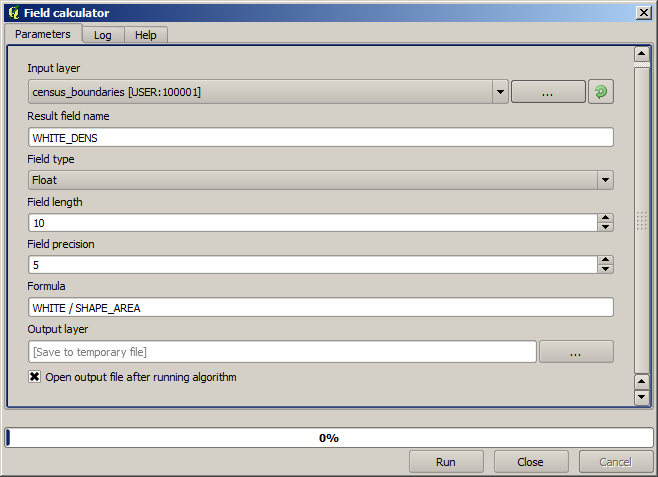This will generate a new field named WHITE_DENS

Now let’s calculate the ratio between the MALES and FEMALES fields to create a new one that indicates if male population is predominant over female population.

Entre a fórmula seguinte

```float(MALES) / FEMALES
```

This time the parameters window should look like this before pressing the OK button.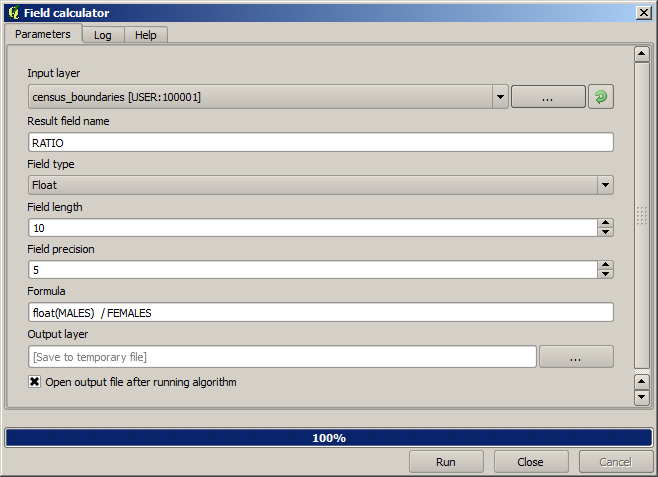Both fields are of type integer, and the result would be truncate to an integer. That’s why we have added the float() function, to indicate that we want floating point number a result. You can use other Python functions as needed, since the calculator supports python commands (the raster calculator, however, doesn’t).

Since the formula field accepts Python syntax, we can have a new field with male or female text strings instead of those ratio value, using the following formula instead.

```'male' if MALES > FEMALES else 'female'
```

The parameters windows should look like this.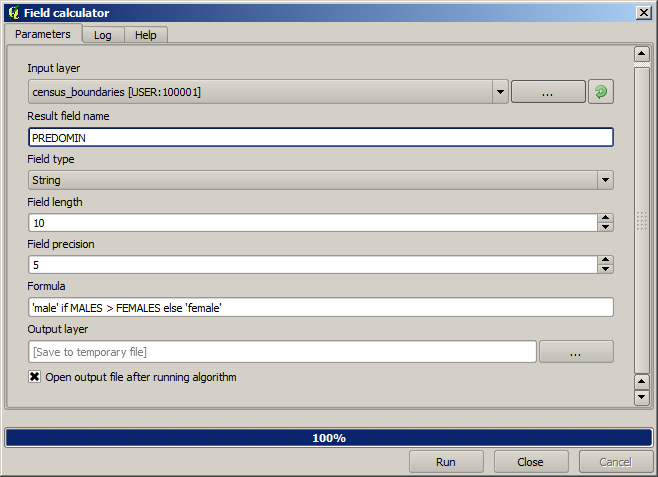A more powerful python field calculator is available in the “Advanced Python field calculator”, which will not be detailed here